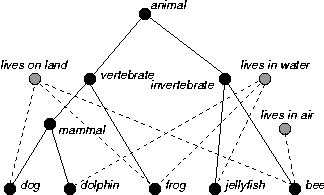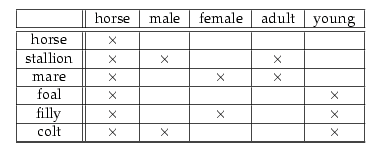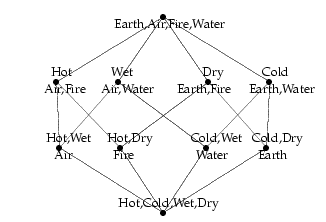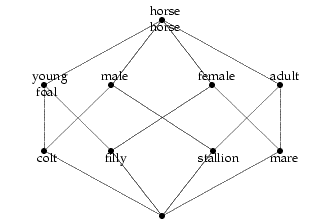# Chapter 8. Concept Lattices: Binding Everything Loosely Together

This is the final chapter in our story, and it explains how many of the mathematical systems we have encountered (hierarchies, vector spaces, and logic) can be viewed as special examples of a space called a lattice.

Much of the mathematics used in this book so far been rooted in developments during a critical period in the middle of the nineteenth century. Chapter 3 described the taxonomic approach to classifying concepts, through the work of Charles Darwin and beyond to the towering figure of Aristotle. The chapters on vectors introduced the geometric breakthroughs of Hamilton and Grassmann, themselves influenced by the work of Descartes. Chapter 7 also described the logic of Boole, which owes much both to Aristotelian logic and Cartesian algebra and geometry. These contributions, sharing many of the same roots, have enabled us to explore concepts using several exciting and diverse methods.

This process of branching out is very important and healthy --- ideas that germinate in one field often bring insight, provoke questions and take on new form in completely different fields. But diversification can also lead to fragmentation, and some of the most important scientific advances have happened when scholars realized that ideas couched in very different terminology could actually be described by a single unified model. The analytic geometry of Descartes (Section 5.3), for example, enabled problems in geometry (such as finding the points where lines and curves intersect) to be solved using methods from algebra (by describing said lines and curves by equations representing the relationships between their x and y coordinates, and solving these equations to find the numerical coordinates).

Broadening this unification into the physical sciences, Isaac Newton went on to represent time as a coordinate, using equations of motion to predict where a particle would be as a function of time. The accurate laws that had been formulated by Galileo Galilei (1564--1642) in the earthly realm and by Johannes Kepler (1571--1630) in the heavenly spheres could then be understood as manifestations of the same universal law of gravitation, which states that every object attracts every other object. More recently, the apparent incompatibility between quantum theory and general relativity (relativity is local, quantum theory non-local; relativity is deterministic, quantum theory non-deterministic) has led physicists beginning with Einstein to search for an underlying Grand Unified Theory.

Our final chapter is about a distinctly twentieth century branch of mathematics that weaves our previous threads together, treating the hierarchies of Aristotle and Darwin, the geometry of Hamilton and Grassmann, and the logic of Boole as different variants of one underlying structure called a lattice. In a way, this is fitting example for the historical process of divergence and convergence we have described: the distinctive property of a lattice is that any two elements can be disjoined (allowed to spread out and diversify) and conjoined (woven together). All this talk of twentieth-century mathematics and unifying theories should not put you off: lattices are not particularly complicated, and like many of the newest branches of scholarship, the ideas behind lattice theory go back at least to classical Greece, and beyond this to roots in astrology and mysticism lost beyond antiquity. We will navigate through this chapter by considering the different ingredients of a lattice in turn, starting with ordered sets (Section 8,1), examples of which include numbers, trees or hierarchies, and some parts of Aristotelean logic. Section 8.2 explains the join and meet operations that make an ordered set into a lattice, examples of which include numbers, lattices of sets, and vector spaces. Section 8.3 introduces the theory of Concept Lattices (Ganter and Wille, 1999), which can be used to describe both the way Aristotle portrays the four elements and the way a modern interlingua (that of Janssen, 2002) represents words for kinds of horse, both applications giving strikingly similar models. Other examples show how the lattice operations give the lowest common multiple and highest common factors in whole number lattices, and eventually the disjunction (OR) and conjunction (AND) operations in logic. Finally, Section 8.4 surveys some of the opportunities for defining a suitable negation or complementation operation on a lattice, again using vector spaces and set theory as examples. This property distinguishes those lattices which can be thought of as logics, the last kind of space we will define axiomatically in this book.A diagram of an ordered set, showing that if we allow multiple inheritance than we can represent information about habitat as well as information about species and genera.

## Sections

### 1. Ordered Sets and Implication

An ordered set is a generalization of a taxonomy or hierarchy. It consists of a collection of objects and relationships between them. These relationships must be transitive (allowing you to form chains of reasoning) and antisymmetric (so never circular). Trees and hierarchies are naturally ordered sets, but they are very restricted ordered sets where each object is only allowed to have one parent. If we allow an object to have multiple parents, we can model much richer structures, giving contextual as well as taxonomic information..
Logic is an important example of the use of ordered sets, and will be returned to often in this chapter. In logic, the ordering on concepts or propositions is derived from the concept of implication. Aristotle's logic is the earliest well-documented example, and its basic properties are described. In particular, Aristotle noted explicitly that logical implication should be transitive (if A implies B and B implies C then A implies C) and never circular.

### 2. Lattices in Mathematics

A lattice is an ordered set equipped with 2 extra operators: a least upper bound or meet and a greatest lower bound or join.

A beautiful example of a lattice is depicted below, in the diagram that shows all the factors of the number 60 (that is, whole numbers that exactly divide 60). This collection forms an ordered set, where A is subsumed by B if and only if A is a factor of B. The meet of two numbers in this lattice is their highest common factor, and the join of two numbers is their lowest common multiple. You can easily find meets and joins by tracing lines up and down from the numbers you're interested in.Lattice showing the factors of 60 and their relationships. To find the lowest common multiple of 2 and 3, follow the paths up which join at the 6 node. To find the highest common factor of 20 and 15, follow that paths down which meet at the 5 node.

There are many important and interesting examples. In logic, the meet of A and B is the conjunction A AND B, because it is the most general concept that is implied by both of A and B. The join of A and B is the disjunction A OR B, because it is the most specific concept that implies both A and B.

Set theory and vector spaces are also examples of lattices, and we will see that they are the underlying lattices of different brands of logic. In set theory, the join of two sets is their set union, and the meet of two sets is their set intersection. In a vector space, the join of two subspaces is the smallest subspace that contains both of them, and the meet is the largest subspace contained in both of them. The meet is the same as with set theory - it is just their intersection. The join is however more general than in set theory, because the join of two lines is not a cross-shape (the union of two lines) but the plane containing them both (the cross-shape plus all the lines joining any two points on the cross-shape).

### 3. Concept Lattices --- Elements and Features

What does any of this have to do with linguistics? Well, we have already seen in Chapter 7 that the differences between Boolean logic (based on set theory) and quantum logic (based on vector spaces) lead search engines with very different though complementary properties.

However, there are deeper and older connections between lattice theory, language, and modelling concepts in the real world. One way of using lattice theory in this fashion is called Formal Concept Analysis, a technique that has become a significant branch of mathematics and information science in its own right over recent decades. The idea behind Formal Concept Analysis is that every concept is represented by the following two things:

• An intent, which is the set of attributes possessed by a concept.
• An extent, which is the set of objects that are examples of this concept.
For example, the intent of the concept dog includes being furry, a companion, having four legs and enjoying going for walkies. The extent of the concept dog includes the examples of my dog Rolo and my parent's dog Plato. (Arguably not all dogs possess the above properties, e.g., a few dogs get by just fine with three legs, but this in general the properties we have stated are reasonably accurate.)

This section of the book describes how you can used this information to build lattices of concepts. Two example lattices are shown in Figure 8.12. They include Janssen's example of kinds of horse, and a much more encient example, which is given by Aristotle's description of the four elements earth, air, fire and water in his treatise On Generation and Corruption. The structures described by Aristotle and Janssen are mathematically identical! The diagrams were drawn using Maarten Janssen's online Java Lattice Building Algorithm, which he designed as part of a technique for building modern interlingua.

 Table 8.1: Cross-table showing the four elements and their attributes. Table 8.1: Cross-table showing types of horse and their attributes.Lattice of the Four Elements and their attributes Lattice of kinds of Horse Figure 8.12: Concept Lattices of the Four Elements (according to Aristotle) and words for kinds of Horses (according to Janssen, 2001) show identical structures.

Such lattices enable us to answer questions such as:

• Q. What do you know about a horse that is either a colt or a stallion? A. It's male.
• Q. What do you know about a horse that is both female and young? A. It's a filly.
In this way, lattices can be used to model the traditional AND and OR operators of logic, and in fact, being a lattice of this sort is part of the modern mathematical definition of being a logic.

### 4. The Axioms of Logic --- Lattices with Negation

We've got implication, AND, and OR, so we only need NOT or complementation and we've got a whole logic. This section described the notion of complementation and expliains how it is used to model negation in logic. The process gives different structures for Boolean logic / set theory and quantum logic / vector spaces. And we discover that the tools we have developed, though 20th century in practice, were defined by Grassmann in 1862. This concludes the formal material of the book.
 Up to Geometry and Meaning | Back to Chapter 7 | On to Conclusion